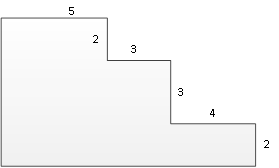### Sample Problem

Find the area of the figure, given that all angles are right.#### Solution

We can cut the whole figure into several rectangular figures, as shown.The area of the top rectangle is just 5 × 2 = 10. The middle rectangle has a length of 5 + 3 = 8 and a width of 3 so it has an area of 8 × 3 = 24. The bottom rectangle has a length of 5 + 3 + 4 = 12 and a width of 2 so the area is 12 × 2 = 24. Thus, the whole area is 10 + 24 + 24 = 58.